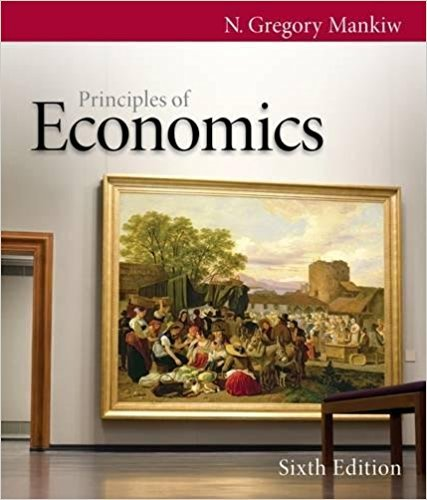×
Get Full Access to Principles Of Economics - 6 Edition - Chapter 30 - Problem Questions for review 30.3
Get Full Access to Principles Of Economics - 6 Edition - Chapter 30 - Problem Questions for review 30.3

×

# Explain the difference between nominal and real variables and give two examples of eachISBN: 9780538453059 472

## Solution for problem Questions for Review 30.3 Chapter 30

Principles of Economics | 6th Edition

• Textbook Solutions
• 2901 Step-by-step solutions solved by professors and subject experts
• Get 24/7 help from StudySoup virtual teaching assistantsPrinciples of Economics | 6th Edition

4 5 1 277 Reviews
19
0
Problem Questions for Review 30.3

Explain the difference between nominal and real variables and give two examples of each. According to the principle of monetary neutrality, which variables are affected by changes in the quantity of money?

Step-by-Step Solution:
Step 1 of 3

Portfolio Management for CFA Session 03 1 Portfolio Risk and Return: Part I Master 1 AFI/CFA Skema Business School Professeur Sébastien DEREEPER LOS a: Calculate and interpret major return measures and describe their appropriate uses. 2 Holding period return is used to measure an investment's return over a specific period. Arithmetic mean return is the simple average of a series of periodic returns. Geometric mean return is a compound annual rate. Money-weighted rate of return is the IRR calculated using periodic cash flows into and out of an account and is the discount rate that makes the present value of cash inflows equal to the present value of cash outflows. Gross retur

Step 2 of 3

Step 3 of 3

##### ISBN: 9780538453059

Unlock Textbook Solution